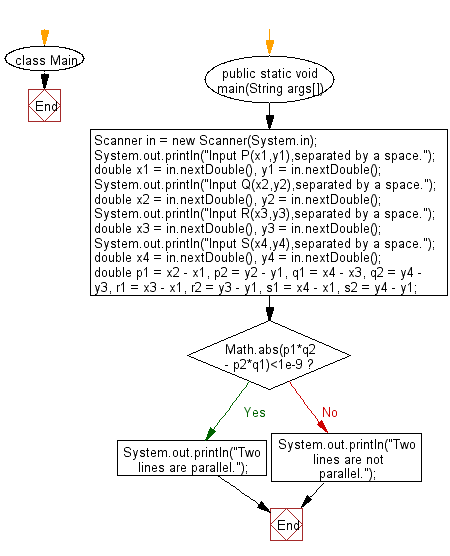# Java Exercises: Test whether two lines PQ and RS are parallel

## Java Basic: Exercise-222 with Solution

Write a Java program to test whether two lines PQ and RS are parallel. The four points are P(x1, y1), Q(x2, y2), R(x3, y3), S(x4, y4).

Input:
−100 ≤ x1, y1, x2, y2, x3, y3, x4, y4 ≤ 100
Each value is a real number with at most 5 digits after the decimal point.

Sample Solution:

Java Code:

`````` import java.util.*;

class Main {

public static void main(String args[]){
Scanner in = new Scanner(System.in);
System.out.println("Input P(x1,y1),separated by a space.");
double x1 = in.nextDouble(), y1 = in.nextDouble();
System.out.println("Input Q(x2,y2),separated by a space.");
double x2 = in.nextDouble(), y2 = in.nextDouble();
System.out.println("Input R(x3,y3),separated by a space.");
double x3 = in.nextDouble(), y3 = in.nextDouble();
System.out.println("Input S(x4,y4),separated by a space.");
double x4 = in.nextDouble(), y4 = in.nextDouble();
double p1 = x2 - x1, p2 = y2 - y1, q1 = x4 - x3, q2 = y4 - y3,
r1 = x3 - x1, r2 = y3 - y1, s1 = x4 - x1, s2 = y4 - y1;
if(Math.abs(p1*q2 - p2*q1)<1e-9)
System.out.println("Two lines are parallel.");
else
System.out.println("Two lines are not parallel.");
}
}
```
```

Sample Output:

```Input P(x1,y1),separated by a space.
5 6
Input Q(x2,y2),separated by a space.
4 2
Input R(x3,y3),separated by a space.
5 3
Input S(x4,y4),separated by a space.
5 6
Two lines are not parallel.
```

Flowchart:Java Code Editor:

What is the difficulty level of this exercise?

﻿

## Java: Tips of the Day

Count the number of occurrences of a char in a String:

```int count = StringUtils.countMatches("a.b.c.d", ".");
```

Ref: https://bit.ly/2OTy6sG